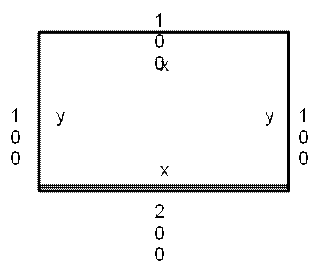# CAT 2021 Question Paper | Quant Slot 3

###### CAT Previous Year Paper | CAT Quant Questions | Question 19

CAT 2021 Quant was dominated by Arithmetic followed by Algebra. In Arithmetic, the questions were dominated by topics like Speed-time-distance, Mixture and Alligations. This year, there was a surprise. The questions from Geometry were relatively on the lower side as compared to the previous years. There were 8 TITA Qs this year. Overall this section was at a medium level of difficulty.

Question 19 : The cost of fencing a rectangular plot is ₹ 200 per ft along one side, and ₹ 100 per ft along the three other sides. If the area of the rectangular plot is 60000 sq. ft, then the lowest possible cost of fencing all four sides, in INR, is

1. 90000
2. 160000
3. 120000
4. 100000

## Best CAT Coaching in Chennai

#### CAT Coaching in Chennai - CAT 2022Limited Seats Available - Register Now!Let the length be x and the breadth be y,
Then the area of the region will be
x×y = 60000
Then the cost of fencing the region will be
200x + 100x + 100y + 100y
300x + 200y

Now we know that the Arithmetic mean ≥ Geometric mean.
So,
$$frac { 300 x + 200 y } { 2 }$≥ $$sqrt [ 2 ] { 300 x \times 200 y }$ 300x + 200y ≥ 2 $\sqrt [ 2 ] { 300 x \times 200 y }$ 300x + 200y ≥ 2 $\sqrt [ 2 ] { 300 \times 200 \times x y }$ 300x + 200y ≥ 2 $\sqrt [ 2 ] { 300 \times 200 \times 60000 }$ 300x + 200y ≥ 2 $\sqrt [ 2 ] { 60000 \times 60000 }$ 300x + 200y ≥ 2$60000$
300x + 200y ≥ 120000)
So we can say the cost will always be greater than 120000

Choice C is the correct answer.

###### CAT Coaching in ChennaiCAT 2023

Classroom Batches Starting Now! @Gopalapuram

###### Best CAT Coaching in Chennai Introductory offer of 5000/-

Attend a Demo Class

##### Where is 2IIM located?

2IIM Online CAT Coaching
A Fermat Education Initiative,
58/16, Indira Gandhi Street,
Kaveri Rangan Nagar, Saligramam, Chennai 600 093

##### How to reach 2IIM?

Mobile: (91) 99626 48484 / 94459 38484
WhatsApp: WhatsApp Now
Email: info@2iim.com